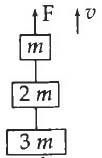#### Three blocks with masses m, 2 m and 3 m are connected by strings, as shown in the figure. After an upward force F is applied on block m, the masses move upward at constant speed. What is the net force on the block of mass 2m?(g is the acceleration due to gravity)Option 1) 6 mg Option 2) zero Option 3) 2 mg Option 4) 3 mgAs we learnt in

Lift is moving down with a = g -- wherein

Apparent weight = 0 (weightlessness)All the blocks are moving with constant velocity. so Fnet = 0.

Correct option is 2.

Option 1)

6 mg

Incorrect

Option 2)

zero

Correct

Option 3)

2 mg

Incorrect

Option 4)

3 mg

Incorrect